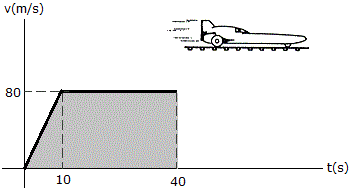Engineering Mechanics - Kinematics of Particle (KOP) - Discussion

Discussion :: Kinematics of Particle (KOP) - General Questions (Q.No.8)

8.From experimental data, the motion of a jet plane while traveling along a runway is defined by the v-t graph shown. Find the position s and the acceleration a when t = 40 s.

 [A]. s = 2.80 km, a = 2.00 m/s2 [B]. s = 2.80 km, a = 0 [C]. s = 2.80 km, a = 2.67 m/s2 [D]. s = 2.80 km, a = 8.37 m/s2

Explanation:

No answer description available for this question.

 Hummer said: (Jun 9, 2012) a= 0 because const speed

 Parhta said: (Aug 24, 2013) What you do is you have to control your hand first without typing?

 Rajkiran Hatheshwar said: (Dec 6, 2015) FIRSTLY AS V=CONST THEN a = 0; NOW FOR's WE KNOW THAT AREA UNDER v&T gives distance change. So that (10*80)/2 + 30*80 = 2.8km.

 Niranjan said: (Feb 14, 2017) Firstly as V=CONST then a = 0; Now for 's' we know that area under v & T gives distance change. So, that (10 * 80)/2 + 30 * 80 = 2.8 km.

 Myra said: (Mar 16, 2018) But the velocity has increased to an extent and so acceleration should have increased before becoming constant. Shouldn't that still be the acceleration at 40s.